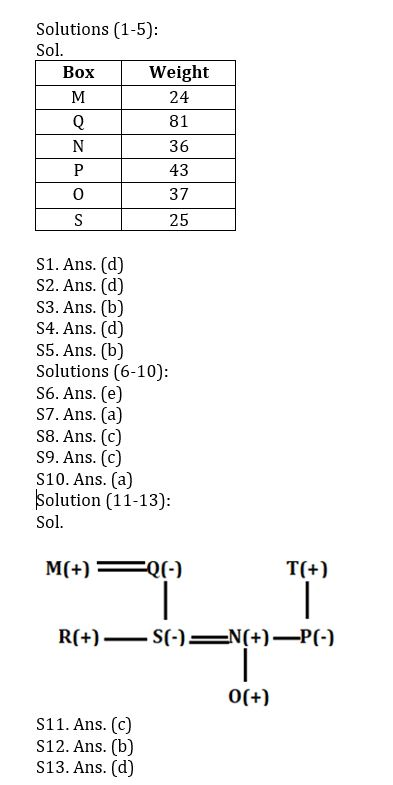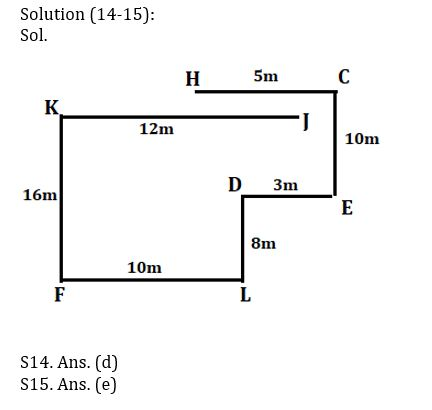Latest Banking jobs   »   Reasoning Ability Quiz For IBPS Clerk...

# Reasoning Ability Quiz For IBPS Clerk Prelims 2022- 31th July

Six boxes M, N, O, P, Q, and S are placed one above another, also they are of different weights (in kg.) i.e., 24, 25, 36, 37, 43, and 81(but not necessarily in the same order). Box P’s weight is not a lowest among all the boxes. M is placed either top or bottom. The weight of the box which is placed at bottom is 25kg. Only one box is placed between P and S. Box Q is not placed just above and below P. Box O is placed below Q but not just below Q. The weight of the box which is placed just above N is a perfect square of an odd number. Box O’s weight is a prime number. Box N is not placed above M whose weight is not a perfect square. Only 2 boxes are placed between M and P whose weight is a prime number. Box P is not third heaviest box.

Q1. What is the weight of Box O?

(a) 24

(b) 25

(c) 36

(d) 37

(d) None of these

Q2. Which of the following box is placed at top?

(a) N

(b) S

(c) O

(d) M

(d) None of these

Q3. How many boxes are placed between Q and the box which weight’s is 25?

(a) One

(b) Three

(c) Two

(d) More than three

(d) None of these

Q4. Which of following box is 24kg?

(a) P

(b) N

(c) Q

(d) M

(e) None of these

Q5. What is the weight of the box which is placed immediate below N?

(a) 36

(b) 43

(c) 24

(d) 81

(e) None of these

Directions (6-10):  Study the following arrangement to answer the given questions

U 3 K % S I \$ V 8 E 5 G © O 4 P @ B 7 Z # 6 & N * 9 R A 4 1 X

Q6. Which of the following element is third to the left of the ninth to the right of ‘O’?

(a) 6

(b) #

(c) 9

(d) Q

(e) None of these.

Q7. Which of the following element is the eighth to the right of the fourteenth from the left end of the above arrangement?

(a) 6.

(b) #

(c) 9

(d) Q

(e) None of these

Q8. How many such numbers are there in the above arrangement, each of which is immediately preceded by a vowel and immediately followed by a consonant?

(a) One

(b) Two

(c) Three.

(d) More than four

(e) None

Q9. What should come next in the following series based on the above arrangement?

(a) U3K

(b) 6*A

(c) 6*R.

(d) 691

(e) &6N

Q10. If we insert “T” after every third letter in the above series starting from the left end of the series, then what will be the 19th element from the right end of the series?

(a) B.

(b) T

(c) @

(d) 7

(e) None of these

Directions (11-13): Study the following information carefully and answer the questions given below:

There are eight members i.e., M, N, O, P, Q, R, S and T who belong to a family having three generation.

N and P are siblings. P is the only daughter of T and she is unmarried. O is the grandson of Q. T is the father-in-law of S. R is the son of M who is husband of Q. R is the brother of S.

Q11. How is N related to O?

(a) Brother

(b) Son

(c) Father

(d) Mother

(e) None of these

Q12. Who among the following is the maternal grandfather of O?

(a) T

(b) M

(c) Q

(d) R

(e) Can’t be determined

Q13. How S related to T?

(a) Son

(b) Daughter

(c) Son-in-law

(d) Daughter-in-law

(e) None of these

Direction (14-15): Study the given information and answer the following question.

Point J is 12m east of point K. Point H is 5m west of point C. Point L is 10m east of Point F. Point F is 16m south of point K. Point D is 8m north of point L. Point E is 3m east of point D. Point C is 10m north of point E.

Q14. What is the shortest distance between H and D?

(a) 10m

(b) 11m

(c) 9m

(d) √104  m

(e) None of these

Q15. Point L is in which direction with respect to C?

(a) South

(b) North-East

(c) East

(d) North-West

(e) South-West

Solutions#### Congratulations!